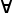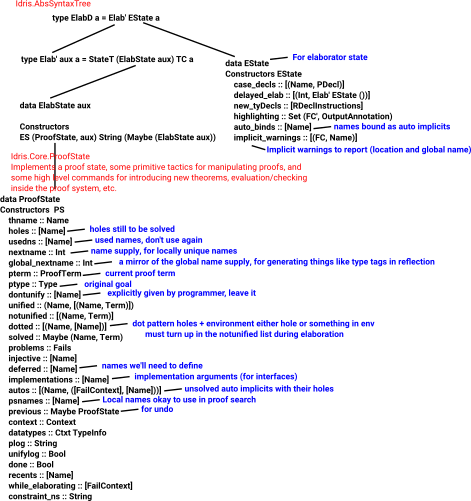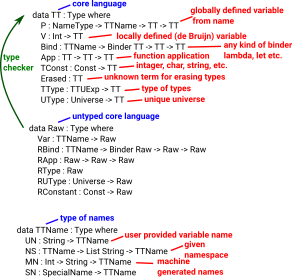# Elaborator Reflection in Idris

 Elaborator Reflection allows user to write meta-programs. These are programs which generate programs.Idris uses a 'proof-theory-like' tactics language to convert Idris programs into a simpler type-theory known as TT syntax.Elaborator reflection allows this language to convert meta programs written by users into TT syntax.

### TT syntax

```Terms,
t ::= c   (constant)
| x       (variable)
| b.t     (binding)
| t t     (application)
| T       (type constructor)
| D       (data constructor)

Binders,
b::= λ x:t     (abstraction)
| let x->t :t   (let binding)
|x :t      (function space)

Constants,
c::= Typei          (type universes)
| i                 (integer literal)
| str               (string literal)```

This is an example, from the paper of how Idris is elaborated into TT.

Idris TT
```vAdd : Num a => Vect n a ->
Vect n a -> Vect n a
vAdd (x :: xs) (y :: ys) =
x + y :: vAdd xs ys```
```vAdd : (a : Type)->(n:Nat)->Num a->
Vect n a->Vect n a->Vect n a
var a:Type , c:Num a.
vAdd a Z c (Nil a) (Nil a)->Nil a
var a:Type , k:Nat , c:Num a,
x:a , xs:Vect k a , y:a , ys:Vect k a.
vAdd a (S k) c ((::) a k x xs) ((::) a k y ys)
-> ((::) a k ((+) c x y) (vAdd a k c xs ys))```

## Tactics

### Forward Deduction

We sometimes think of a deduction rule as starting from a set of things we know (premises) and leading to something we wish to prove (conclusion).### Backward Deduction (Tactics)

When we are using a tactics system we start with what we want to prove (conclusion) and we go back to all the things we have to prove for that to be true (the premises).So in a tactics system we start with a goal (conclusion), then we reduce this to a set of sub-goals and so on.

### Proof Tree

The proof tree can hold the tree of goals and sub-goals encoded as proof objects.

The complete proof tree can hold a theorem (or by Curry-Howard a program).

In proof assistants the proof tree is constructed, from an incomplete tree, by filling in the holes (unknown terms).

The nodes can be encoded as λ-terms or a collection of tactic calls. However λ-terms are not very good at holding holes (open terms) because they require extra (outside proof) information.Add monadic structure to proof object to allow exra information to be held for holes.

https://github.com/idris-lang/Idris-dev/blob/master/libs/prelude/Language/Reflection/Elab.idr

A tactic is impemented by a function

TT -> List (TTName, TT) -> Tactic

where

• The first argument is the goal type,
• The second is the local proof context,
• The return result

## Proof State

A proof state is a tuple (see Brady 2013) consisting of the following:

• A global context, C, containing pattern matching definitions and their types.
• A local context, Δ, containing pattern bindings.
• A proof term, e, in TTdev
• Unsolved unification problems, P
• These take the form ( Γ,e1,e2), i.e. the context in which the problem is to be solved, and the expressions to be unified.
• A hole queue, Q.

## Identity Proofsee here

## Idris Top LevelThe Elab monad holds a proof state inside, which has:

• A goal type
• A proof term that is incrementally built up.
• A hole queue
• A collection of open unification problems
• A supply of fresh names

The following is Idris code from module Language.Reflection.Elab which is in libs->prelude.The state is really held in the Haskell code. see Module : Idris.Elab.Term Description : Code to elaborate terms. ``` -- | Do a step in the reflected elaborator monad. The input is the -- step, the output is the (reflected) term returned. runTacTm :: Term -> ElabD Term runTacTm tac@(unApply -> (P _ n _, args)) | n == tacN "Prim__Solve" = do ~[] <- tacTmArgs 0 tac args -- patterns are irrefutable -- because `tacTmArgs` returns lists of exactly the size -- given to it as first argument solve returnUnit | n == tacN "Prim__Goal" = do ~[] <- tacTmArgs 0 tac args hs <- get_holes ```### Elaborator Code

 Running elaborator from within Idris environment: ```idNat : Nat -> Nat idNat = %runElab (do intro `{{x}} fill (Var `{{x}}) solve)```

This uses the %runElab e declaration.

'e' has the type Elab ()

• new type declation function
• data type definitions

generated by the Elab action generated by e to the context.https://koerbitz.me/posts/A-Look-at-the-Idris-Internals-Part-I-Overview-and-Parsing.html```Idris> :browse Language.Reflection.Elab.Tactics Namespaces: Names: addImplementation : TTName -> TTName -> Elab () apply : Raw -> List Bool -> Elab (List TTName) attack : Elab () check : List (TTName, Binder TT) -> Raw -> Elab (TT, TT) claim : TTName -> Raw -> Elab () compute : Elab () converts : TT -> TT -> Elab () convertsInEnv : List (TTName, Binder TT) -> TT -> TT -> Elab () currentNamespace : Elab (List String) debug : Elab a debugMessage : List ErrorReportPart -> Elab a declareDatatype : TyDecl -> Elab () declareType : TyDecl -> Elab () defineDatatype : DataDefn -> Elab () defineFunction : FunDefn Raw -> Elab () fail : List ErrorReportPart -> Elab a fill : Raw -> Elab () focus : TTName -> Elab () forall : TTName -> Raw -> Elab () gensym : String -> Elab TTName getEnv : Elab (List (TTName, Binder TT)) getGoal : Elab (TTName, TT) getGuess : Elab TT getHoles : Elab (List TTName) getSourceLocation : Elab SourceLocation intro : TTName -> Elab () intro' : Elab () isTCName : TTName -> Elab Bool letbind : TTName -> Raw -> Raw -> Elab () lookupArgs : TTName -> Elab (List (TTName, List FunArg, Raw)) lookupArgsExact : TTName -> Elab (TTName, List FunArg, Raw) lookupDatatype : TTName -> Elab (List Datatype) lookupDatatypeExact : TTName -> Elab Datatype lookupFunDefn : TTName -> Elab (List (FunDefn TT)) lookupFunDefnExact : TTName -> Elab (FunDefn TT) lookupTy : TTName -> Elab (List (TTName, NameType, TT)) lookupTyExact : TTName -> Elab (TTName, NameType, TT) matchApply : Raw -> List Bool -> Elab (List TTName) metavar : TTName -> Elab () normalise : List (TTName, Binder TT) -> TT -> Elab TT operatorFixity : String -> Elab Fixity patbind : TTName -> Elab () patvar : TTName -> Elab () resolveTC : TTName -> Elab () rewriteWith : Raw -> Elab () runElab : Raw -> Elab () -> Elab (TT, TT) search : Elab () search' : Int -> List TTName -> Elab () solve : Elab () sourceLocation : Elab () unfocus : TTName -> Elab () whnf : TT -> Elab TT Idris> ```